# Second order differential equations with an irregular singularity at the origin and a large parameter: convergent and asymptotic expansions

### Abstractcarpathian_2016_32_1_063_070_abstract

### Full PDFcarpathian_2016_32_1_063_070

We consider the second order linear differential equation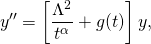where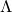is a large complex parameter and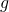is a continuous function. In previous works we have considered the case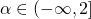and designed a convergent and asymptotic method for the solution of the corresponding initial value problem with data at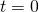. In this paper we complete the research initiated in those works and analyze the remaining case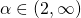. We use here the same fixed point technique; the main difference is that forthe convergence of the method requires that the initial datum is given at a point different from the origin; for convenience we choose the point at the infinity. We obtain a sequence of functions that converges to the unique solution of the problem. This sequence has also the property of being an asymptotic expansion for large(not of Poincar\’e-type) of the solution of the problem. The generalization to non-linear problems is straightforward. An application to a quantum mechanical problem is given as an illustration.